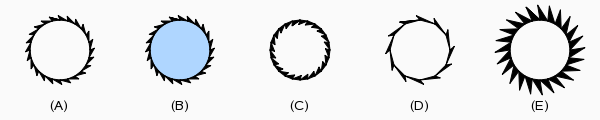# OEDrawSawCircle¶

bool OEDrawSawCircle(OEDepict::OEImageBase &image,
const OEDepict::OEPen &pen,
unsigned int patternDirection=OEPatternDirection::Outside,
double patternAngle=15.0, double patternWidthRatio=0.15)


Draws a circle with the OECircleStyle_Saw style.OEDrawSawCircle

image

The image on which the circle is drawn.

center

The center of the circle.

pen

The graphical properties of the circle.

See examples (A) and (B) in Figure: OEDrawSawCircle.

patternDirection

Specifies whether the pattern is drawn inside or outside of the circle. This value has to be from the OEPatternDirection namespace.

See example (C) in Figure: OEDrawSawCircle.

patternAngle

Specifies the angle (in degrees) between the patterns of the circle. The angle has to be in a range of $$[1.0^{\circ} - 90.0^{\circ}]$$

See example (D) in Figure: OEDrawSawCircle.

patternWidthRatio

Specifies the width of the pattern of the circle relative to its radius. The ratio has to be in a range of $$[0.01 - 1.00]$$.

See example (E) in Figure: OEDrawSawCircle.